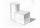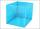# Cube + expression of a variable from formula - problems

1. Two cubesThe surfaces of two cubes, one of which has an edge of 22 cm longer than the second are differ by 19272 cm2. Calculate the edge length of both cubes.
2. Cube in a sphereThe cube is inscribed in a sphere with volume 6116 cm3. Determine the length of the edges of a cube.
3. TerezaThe cube has area of base 256 mm2. Calculate the edge length, volume and area of its surface.
4. Cube wallCalculate the cube's diagonal diagonal if you know that the surface of one wall is equal to 36 centimeters square. Please also calculate its volume.
5. Pyramid in cubeIn a cube with edge 12 dm long we have inscribed pyramid with the apex at the center of the upper wall of the cube. Calculate the volume and surface area of the pyramid.
6. Cube 5The content area of one cube wall is 32 square centimeters. Determine the length of its edges, its surface and volume.
7. Sugar cubesThe glass has 600 ml of tea, which represents 80% of the volume of the glass. If you put twenty regular sugar cubes of 2 cm in the tea, how many ml of tea are poured?
8. Cube wallThe perimeter of one cube wall is 120 meters. Calculate the surface area and the body diagonal of this cube.
9. Surface area of cylinderDetermine the lateral surface of the rotary cylinder which is circumscribed cube with edge length 5 cm.
10. Cube diagonalsDetermine the volume and surface area of the cube if you know the length of the body diagonal u = 216 cm.
11. Cube 5The surface of the cube is 15.36 dm2. How will change the surface area of this cube if the length of the edges is reduced by 2 cm?
12. Cube 8The surface of the cube is 0.54 m2. Calculate the length of the cube edge.
13. Cube and sphereCube with the surface area 150 cm2 is described sphere. What is sphere surface?
14. Cube edgeDetermine the edges of the cube when the surface is equal to 37.5 cm square.
15. Wall diagonalCalculate the length of wall diagonal of the cube whose surface is 384 cm square.
16. Cube wallsFind the volume and surface area of the cube if the area of one wall is 40cm2.
17. Cube 1-2-3Calculate the volume and surface area of the cube ABCDEFGH if: a) /AB/ = 4 cm b) perimeter of wall ABCD is 22 cm c) the sum of the lengths of all edges of the cube is 30 cm.
18. Volume and areaWhat is the volume of a cube which has area of 361 cm2?
19. Surface of cubesPeter molded a cuboid 2 cm, 4cm, 9cm of plasticine. Then the plasticine split into two parts in a ratio 1:8. From each part made a cube. In what ratio are the surfaces of these cubes?
20. Cube into sphereThe cube has brushed a sphere as large as possible. Determine how much percent was the waste.

Do you have an interesting mathematical problem that you can't solve it? Enter it, and we can try to solve it.

To this e-mail address, we will reply solution; solved examples are also published here. Please enter e-mail correctly and check whether you don't have a full mailbox.